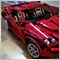# Trouble with coding entry/exit arrows182

Hi,

I'm trying to code my strategy into my indicator so it prints buy/sell arrows that I can easily convert into an EA, however I'm having some troubles coding the logic for it.

Here's what I have coded for it so far:

if (LS)

{

x = 1;

}

if (x==1 && mColor==Green)

{

x =2;

}

if (x==2)

{

TextCreate(0,indicator+IntegerToString(time[i]),0, time[i],low[i]-(distn /(heigh /(33))),"x","Arial",10,clrOrange,0,ANCHOR_CENTER);

x=3;

}

if (longex && x==3)

{

a = 1;

}

if (a==1)

{

TextCreate(0,indicator+IntegerToString(time[i]),0, time[i],low[i]-(distn /(heigh /(33))),"z","Arial",10,clrOrange,0,ANCHOR_CENTER);

a=2;

}

that all works, and what that is doing is saying when i get a long signal (B is printed) I then enter when that MA becomes green (x is printed) and that is all fine. Next, when that white dashed line is equal to price I want a z printed, and that is fine. My problem is I want "k" to be printed on the first bar that isn't touching that dashed white line, as indicated by the orange line in the pic. What I have currently is:

if (a==2 and High != dashedline)

{

TextCreate(0,indicator+IntegerToString(time[i]),0, time[i],low[i]-(distn /(heigh /(33))),"k","Arial",10,clrOrange,0,ANCHOR_CENTER);

a=3;

}

but that condition is never met and k is never printed. Can anyone help me with this?

also, a=0 and x=0 are outside of the oncalculate and oninit methods.

thank you

Files:746

Pierce Daly:

I'm trying to code my strategy into my indicator so it prints buy/sell arrows that I can easily convert into an EA, however I'm having some troubles coding the logic for it.

that all works, and what that is doing is saying when i get a long signal (B is printed) I then enter when that MA becomes green (x is printed) and that is all fine. Next, when that white dashed line is equal to price I want a z printed, and that is fine. My problem is I want "k" to be printed on the first bar that isn't touching that dashed white line, as indicated by the orange line in the pic. What I have currently is:

if (a==2 and High != dashedline)

{

TextCreate(0,indicator+IntegerToString(time[i]),0, time[i],low[i]-(distn /(heigh /(33))),"k","Arial",10,clrOrange,0,ANCHOR_CENTER);

a=3;

}

but that condition is never met and k is never printed. Can anyone help me with this?

also, a=0 and x=0 are outside of the oncalculate and oninit methods.

Doesn't look suspicious, and I assume your 'and' is just a typo here...

So if you need help, you'll need to post more of your code...182

yeah just a typo, so that looks fine???

this is the only portion of my code dealing with the arrows for the EA.746

Pierce Daly:

yeah just a typo, so that looks fine???

this is the only portion of my code dealing with the arrows for the EA.

Because you were able to print 'x' and 'z', so your 'TextCreate()' should also be fine.

Time-series arrays cannot be wrong.

Unless you change your 'a', 'i', 'distn' or 'heigh' in between 'z' and 'k' (which I assume you didn't), and whatever value that dashedline takes should not be equal to High most of the time.

So from the lines that you've shown, nothing seems wrong.182

Seng Joo Thio:

Because you were able to print 'x' and 'z', so your 'TextCreate()' should also be fine.

Time-series arrays cannot be wrong.

Unless you change your 'a', 'i', 'distn' or 'heigh' in between 'z' and 'k' (which I assume you didn't), and whatever value that dashedline takes should not be equal to High most of the time.

So from the lines that you've shown, nothing seems wrong.

thanks, I'll try to look deeper into it